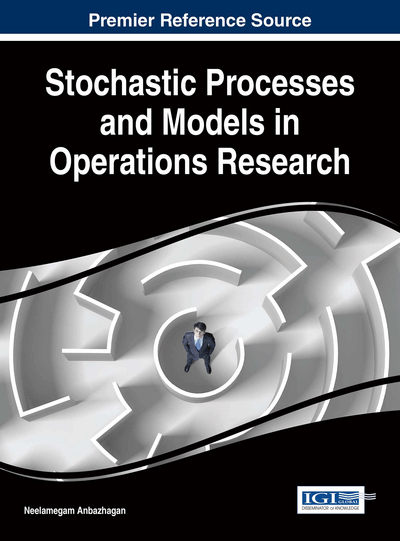# A Method for Analyzing Queues in Fuzzy Environment

Thillaigovindan Natesan (Arba Minch University, Ethiopia)
DOI: 10.4018/978-1-5225-0044-5.ch001

## Abstract

In this chapter a new method for analyzing queues in fuzzy environment is presented. After explaining the new technique, it is applied to a fuzzy bulk queue with modified Bernoulli vacation and restricted admissible customers. Batches of customers arrive at the system according to a compound Poisson process. All arriving batches are not allowed to enter the system. The restriction policy depends on availability or otherwise of the server. This system is analyzed in fuzzy environment using the new method developed. Some special cases are discussed and a numerical study is also carried out. The new method can be applied to any queuing system in fuzzy environment. In this method the input parameters like arrival rate, service rate, vacation rate etc. are described by fuzzy numbers of specific type (triangular, trapezoidal, quadratic, Gaussian etc.) and the system performance measures like average queue size, average waiting time in the queue, average number of customers in the system are all obtained as fuzzy numbers of the same type, which include the crisp solution.
Chapter Preview
Top

## Introduction

In this chapter a new method for analyzing queues in fuzzy environment is presented. This method combines the Zadeh‘s extension principle, the α-cut approach and the parametric non-linear programing technique. The necessary basic concepts in fuzzy theory are explained first. The crisp case of a queuing model considered is described and the corresponding steady state results are presented next. Some special cases of interest are also considered. The same model in fuzzy environment is described subsequently and the new method of analyzing the fuzzy model is explained. An algorithm is developed for this purpose. A method for evaluating the membership functions of interest for the fuzzy case is described and is illustrated with a numerical study. The new method can be applied to any queuing system in fuzzy environment.

In this method the input parameters like arrival rate, service rate, vacation rate etc., are described by fuzzy numbers of specific type (triangular, trapezoidal, quadratic, Gaussian etc.,) and the system performance measures like average queue suze, average waiting time in the queue, average number of customers in the system are all obtained as fuzzy numbers of the same type, which include the crisp solution.